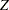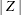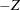Autocorrelation Analysis

You can use the autocorrelation analysis features of PROC VARIOGRAM to compute the autocorrelation Moran’sand Geary’sstatistics and to obtain the Moran scatter plot. In the following statements, you ask for the Moran’sand Geary’sstatistics under the assumption of randomization using binary weights, in addition to the Moran scatter plot:

```proc variogram data=thick outv=outv plots(only)=moran;
compute lagd=7 maxlag=10 autocorr(assum=random);
coordinates xc=East yc=North;
var Thick;
run;
```

For the autocorrelation analysis with binary weights and the Moran scatter plot, the LAGDISTANCE= option indicates that you consider as neighbors of an observation all other observations within the specified distance from it.

Figure 98.8 shows the output from the requested autocorrelation analysis. This includes the observed (computed) Moran’sand Geary’scoefficients, the expected value and standard deviation for each coefficient, the correspondingscore, and the p-value in the Prcolumn. The low p-values suggest strong autocorrelation for both statistics types. A two-sided p-value is reported, which is the probability that the observed coefficient lies farther away fromon either side of the coefficient’s expected value—that is, lower thanor higher than. The sign offor both Moran’sand Geary’scoefficients indicates positive autocorrelation in the Thick data values; see the section Interpretation for more details.

Figure 98.8 Output Table for the Autocorrelation Statistics
 Spatial Correlation Analysis with PROC VARIOGRAM

The VARIOGRAM Procedure
Dependent Variable: Thick

Autocorrelation Statistics
Assumption Coefficient Observed Expected Std Dev Z Pr > |Z|
Randomization Moran's I 0.9240 -0.0244 0.145 6.53 <.0001
Randomization Geary's c 0.0162 1.0000 0.175 -5.62 <.0001

The requested Moran scatter plot is shown in Figure 98.9. The plot includes all nonmissing observations that have neighbors within the specified LAGDISTANCE= distance. The horizontal axis displays the standardized Thick values, and the vertical axis displays the corresponding weighted average of their neighbors. The plot data points are concentrated in the upper right and lower left quadrants defined by the linesand, and clearly around the axes’ diagonal reference lineof slope 1. This fact indicates strong positive spatial association in the thick data set observations. Therefore, for each observation its neighbors within the specified LAGDISTANCE= distance have overall similar Thick values to that observation. The plot also displays the linear regression slope, whose value is the Moran’scoefficient when the binary weights are row-averaged. See the section The Moran Scatter Plot for more details about the Moran scatter plot.

Figure 98.9 Moran Scatter Plot for Coal Seam Thickness Data